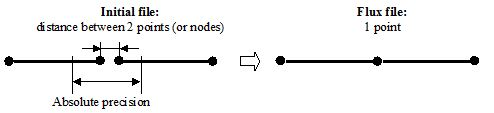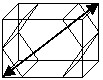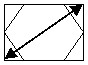# General options for conversion

## Introduction

The general options for conversion available for all formats are the following :

• choose a coordinate system : to place the imported part in the Flux project.
• choose a system unit : to determine dimension units of the part
• choose precision : to define the minimum distance enabling to distinguish two points

These options are detailed in the following blocks.

## Coordinate system

At the moment of importation, a coordinate system is created in the Flux project with the name XXXi (where XXX = extension corresponding to the imported format). This coordinate system coincides with the principal coordinate system XYZ1. Then the user can displace the device (for example, with respect to the infinite box, etc.) by modifying the position of the imported coordinate system.

At the moment of importation, the user can position the device in one of the following coordinate systems:

• the proper coordinate system of the device: XXXi
• a predefined coordinate system : XYZ1, Z_ON_OX, Z_ON_OY
• an user coordinate system: …

## Length unit

The device is described in proper units in the initial file, but the information about the system unit is not present in the import file.

At the moment of importation, the user can choose a length unit as follows:

• by default: meter
• another possibility: meter * conversion factor
Note: The conversion factor is the ratio between the length unit chosen by the user and the Flux length unit, which is the meter.

Examples of conversion are presented in the table below.

If the entities in the initial file are in … and the conversion factor is equal with … the unit in the Flux project is …
Meter 1 Meter
Millimeter 0.001 Millimeter
Micron (micrometer) 10-6 Micron
Attention: The length unit previously chosen is automatically assigned to the imported coordinate system XXXi. If the device is imported in another coordinate system, the user must assure that the length unit of this coordinate system is compatible with the importation length unit.

## Precision

The absolute precision is the minimum distance between two points of the geometry (or between two nodes of the mesh) from which the two points (or the two nodes) of the initial file are represented by only one point in the Flux project.The absolute precision is:

• either imposed by the user
• or automatically computed by Flux (automatic precision)

## Automatic precision

The automatic precision, quantity automatically computed by Flux, is obtained by means of the following formula:

Absolute precision = Relative precision * Diagonal

where:

• Relative precision, also called relative epsilon, is a coefficient independent of the length unit, fixed to 10-5 for the importation
• Diagonal is the distance between two faraway points of the box surrounding the device (see the figure below)
3D geometry 2D geometry